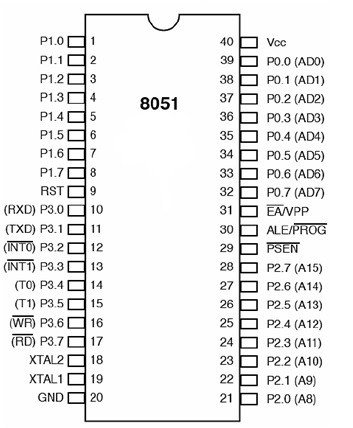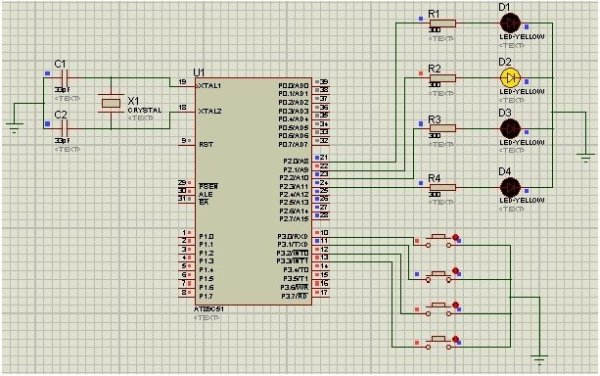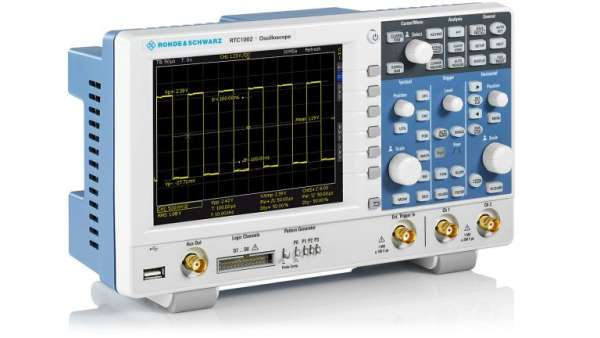# How to use input output ports 8051 microcontroller|LED blinking

How to use input output ports 8051 microcontroller. 8051 microcontroller has 40 pins comprising of four I/O ports. 8 pins are used for specific purposes and 32 pins are used as input/output pins to connect the microcontroller with the peripheral devices. Each of PORT is 8-bit, which can be configured as input or output port. In this article we will learn how to use I/O ports of 8051 microcontroller so that they can be used for read and write purpose. Before reading this article further you should know how to program 8051 microcontroller with Keil.

Page Contents

• 1 PIN DIAGRAM of  input output ports 8051 microcontroller
• 2 PORTS DESCRIPTION of  input output ports 8051 microcontroller
• 3 PIN CONFIGURATION SETTINGS of input output ports 8051 microcontroller
• 4 LED blinking with input output ports 8051 microcontroller
• 5 PROTEUS SIMULATIONS of LED blinking with  input output ports 8051 microcontroller
• 6 Video lecture on input output ports of 8051 microcontroller
• 7 CODE of input output ports 8051 microcontroller

### PIN DIAGRAM of  input output ports 8051 microcontroller### PORTS DESCRIPTION of  input output ports 8051 microcontroller

PORT 0:

P0 includes pins (32-39). It is an I/O port with some alternative functions.

1. When the external memory is used with microcontroller, then the lower address byte (addresses A0-A7) is applied on P0. If not using external memory, all bits of P0 are configured for I/O purposes.
2. P0doesn’t contain built-in pull-up resistor.
• If any pin of this port is configured as an input, then it act as it “floats” and input has unlimited input resistance and undetermined potential.
• When any pin of this port is configured as an output, it acts as an “open drain”. If logic 0 is given to port bit, the pin will be connected to ground (0V). If logic 1 is given to port bit, the external output will keep on “floating”. So, to apply logic 1 (5V) on this output pin, it is necessary to connect an external pull-up resistor.
READ  How to get started with PICAXE 08M microcontroller

PORT 1:

P1 includes pins (1-8). It is an I/O port with no alternative functions and configured only as general I/O purposes. P1 contains built-in pull-up resistor and is compatible with TTL circuits.

PORT 2:

P2 includes pins (21-28). It is an I/O port with alternative functions as port 0 except P1 when the external memory is used with microcontroller, then the higher address byte (addresses A8-A15) is applied on P2. When no external memory is used, all bits of P0 are configured as I/O purposes.

PORT 3:

P3 includes pins (10-17). It is an I/O port with different function. For using the alternative functions, a logic one (1) must be applied to appropriate bit of the P3 register. In hardware terms, this port is similar to P0 but it contains built-in pull-up resistor.

### PIN CONFIGURATION SETTINGS of input output ports 8051 microcontroller

For input:        Pin is configured as 1 for input.

For output:      Pin is configured as 0 for output.

Current limitations:

• When pin is are configured as an output, single pin can receive a current of 10mA.When pin is configured as input, then built-in pull-up resistors provide very weak current, but can handle up to 4 TTL inputs.
• If a port’s all 8 bits are active, then the total current must be limited to 15mA. But for port 0, it can be up to 26mA (port P0: 26mA).
• If all 4 ports (32 bits) are active, then the total current must be limited to 71mA.

### LED blinking with input output ports 8051 microcontroller

In this example I will explain how to use I/O ports of 8051 microcontroller. Input to the microcontroller can be given through push button and output can be seen through LED. Firstly all the port pins are defined. Port 2 is used as output port and port 3 is used as input port.  These Ports are initialized with 0 (to write) and 1 (to read) logic respectively. Delay function is used to give the delay in showing output. Frequency of crystal should be set to 11.059MHz. Program is written in C language using “keil” software and hex file isloaded in the microcontroller.

READ  LED Interfacing with PIC Microcontroller: Embedded C program with circuit using pic microcontoller

WORKING of input output ports 8051 microcontroller:

When we run the simulation, if first push button is pressed, first led will glow. It will keep glowing for 1 second and then it goes off. When 2nd push button is pressed, 2nd led will glow. Any LED can be ON according to the respective input of the button. If we want to show the output for more time then delay call is passed large integer value.

### PROTEUS SIMULATIONS of LED blinking with  input output ports 8051 microcontroller### CODE of input output ports 8051 microcontroller

#include<reg51.h>
sbit Led1 = P2^0;          //Defining LED Pins

sbit Led2 = P2^1;

sbit Led3 = P2^2;

sbit Led4 = P2^3;

sbit Button1 = P3^0;      //Defining Button Pins

sbit Button2 = P3^1;

sbit Button3 = P3^2;

sbit Button4 = P3^3;

void Delay(int);     //Delay function declaration

int main ()

{

P2 = 0x00;                                          //used as output port

P3 = 0xFF;                                          //used as input port

do

{

if(Button1 == 0 )                               //If 1stswitch pressed

{

Led1 = 1;                                        //1st LED ON

Delay(1000);

Led1 = 0;                                        //LED OFF

}

if(Button2 == 0 )               //If 2ndswitch pressed

{

Led2 = 1;                                        //2nd LED ON

Delay(1000);

Led2 = 0;

}

if(Button3 == 0 )               //If 3rdswitch pressed

{

Led3 = 1; //LED ON                    //3rd LED ON

Delay(1000); //Delay

Led3 = 0; //LED OFF

}

if(Button4 == 0 )               //If 4thswitch pressed

{

Led4 = 1; //LED ON                    //4th LED ON

Delay(1000); //Delay

Led4 = 0; //LED OFF

}

}

while(1);

}

void Delay(int k)

{

int j;

inti;

for(i=0;i<k;i++)

{

for(j=0;j<100;j++)

{

}

}

}

How to use input output ports 8051 microcontroller|LED blinking

READ  Spooky Led Lamp using PIC12F675 microcontroller

#### Current Project / Post can also be found using:

• input output of pic16f84a
• PIC16F876A 8*8 matrix display
• pic18 lightup led project